# Vapor Pressure Formula & Example

Maram Ghadban, Nichole Miller
• Author

A freelance tutor equipped with a bachelor's of science in chemical engineering. Graduated from the American University of the Middle East with a GPA of 3.87, performed a number of scientific primary and secondary research. Tutored university level students in various courses in chemical engineering, math, and art. Has experience tutoring middle school and high school level students in science courses.

• Instructor
Nichole Miller

Nichole is a research scientist with a PhD in Materials Science & Engineering.

Learn vapor pressure definition and discover a few common examples which involve vapor pressure. Know and use formulas that involve the use of vapor pressure. Updated: 12/01/2021

Show

## Vapor Pressure Definition

The vapor pressure definition is the pressure exerted by the vapors of a substance in a closed system at equilibrium and a fixed temperature. The pressure exerted by vapors of a substance that is at thermodynamic equilibrium with its solid or liquid is directly proportional to temperature; the vapor pressure increases as the temperature increases. The vapor pressure also decreases as a response to any decrease in the temperature. The reason behind this is related to the relationship between temperature and the kinetic energy of the molecules. A rise in the temperature of a liquid supplies its molecules with energy; this leads to an increase in the kinetic energy of the molecules and the frequency of molecular collisions. Consequently, the number of molecules entering the gas phase increases, which in turn increases the vapor pressure.

What does equilibrium mean? Take a bottle of water as an example of a closed system, where liquid water evaporates and turns into vapor over time. The water vapor trapped in the bottle condenses and returns to the liquid phase. The system is at equilibrium when the amount of water is evaporating is equal to the water that is condensing.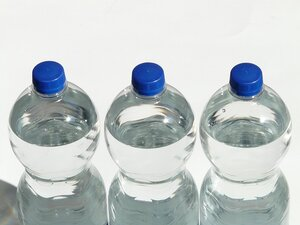### Vapor Pressure Examples

A real-life vapor pressure example is the pressure cooker. It is a closed chamber that traps the vapor and prevents it from escaping to the atmosphere. The pressure cooker works by the following principle: the water in the cooker evaporates and accumulates in the closed chamber. The increased number of vapor molecules consequently increases the vapor. This speeds up the process of cooking the food. When the heat supplied to the cooker is terminated, the vapor condenses and returns to the liquid phase, decreasing the vapor pressure.Boiling tea in a kettle is another example of vapor pressure. When the water in the kettle begins to boil, the number of water molecules transitioning to the vapor phase increase. The vapor molecules exert pressure on the kettle's lid. This can be seen by the moving of the kettle's lid.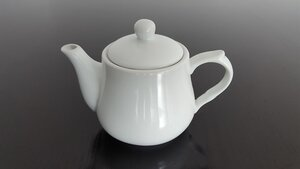## What Is Vapor Pressure?

Water can take the form of a liquid, a solid (ice), or a gas (water vapor). Ice melts to form water, and water evaporates to form water vapor. Think about a water bottle like the one shown here: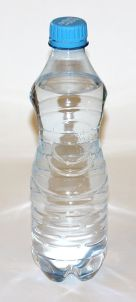What's inside? You can see water and air, but what you may not have realized is that there is also water vapor. In a water bottle, a little bit of the water is constantly evaporating and becoming water vapor. At the same time, some of the water vapor is constantly condensing to become a liquid. At equilibrium, the amount of water evaporating is equal to the amount of water vapor condensing, so the amounts of water and water vapor are constant.

The water vapor exerts a pressure on the water bottle in the same way that air pumped into a tire exerts pressure on the tire. The pressure exerted by the water vapor is the vapor pressure. In more general terms, vapor pressure is the pressure exerted by a gas in equilibrium with the same material in liquid or solid form. Just as distance can be measured in a variety of units (miles, feet, kilometers, etc.), we can also measure vapor pressure with many different units (kPa, atm, bar, mm Hg, torr).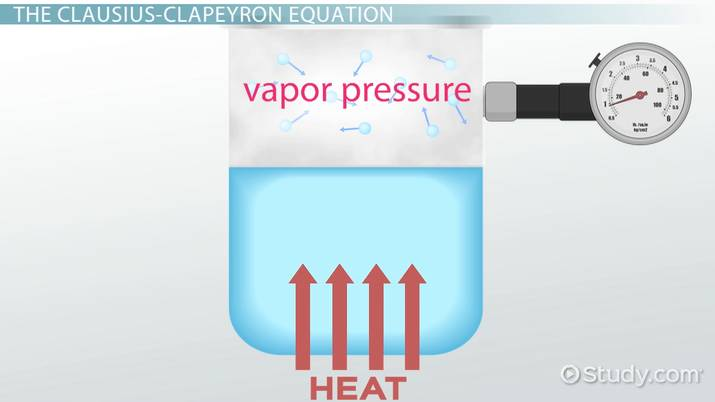An error occurred trying to load this video.

Try refreshing the page, or contact customer support.

Coming up next: What is a Hygrometer? - Definition & Uses

### You're on a roll. Keep up the good work!

Replay
Your next lesson will play in 10 seconds
• 0:03 What is Vapor Pressure?
• 1:17 The Clausius-Clapeyron…
• 2:06 Clausius-Clapeyron…
• 3:19 Raoult's Law
• 4:13 Raoult's Law Example
• 5:27 Lesson Summary
Save Save

Want to watch this again later?

Timeline
Autoplay
Autoplay
Speed Speed

## Vapor Pressure Formula

The first vapor pressure formula is derived from Raoult's Law. It is as follows:

{eq}P_a = x_a P_{0,a} {/eq}

Where:

• {eq}P_a {/eq} is the vapor pressure of species a in a mixture. Alternatively, known as the partial pressure of species a.
• {eq}x_a {/eq} is the molar fraction of species a.
• {eq}P_{0,a} {/eq} is the vapor pressure of pure a.

The units of {eq}P_a {/eq} and {eq}P_{0,a} {/eq} can be any pressure units for long as the same unit is being used for both parameters. The second vapor pressure formula relates the vapor pressure of a pure substance at different temperatures:

{eq}ln\frac{P_1}{P_2}=\frac{H_v}{R}(\frac{1}{T_2}-\frac{1}{T_1}) {/eq}

Where:

• {eq}ln {/eq} is the natural log.
• {eq}P_1 {/eq} is the vapor pressure at the initial temperature {eq}T_1 {/eq}.
• {eq}P_2 {/eq} is the vapor pressure at the final temperature {eq}T_2 {/eq}.
• {eq}H_v {/eq} is the substance's enthalpy of vaporization. Its unit is in kJ/mol.
• R is the universal gas constant. This constant value differs from unit to unit. R = 0.008314 kJ/mol K will be the value used in this lesson.

Note that the temperature must always be in Kelvins. The unit of pressure in this equation will be presented in atm. This is preferable to avoid any unnecessary unit conversion.

### How to Calculate Vapor Pressure?

The vapor pressure equation is derived from Raoult's Law and is used when determining the vapor pressure of a certain species in an ideal liquid mixture. Raoult's Law states that the partial pressure of a species in a vapor is directly proportional to its molar fraction in the ideal mixture. This means that the more of the substance is present as a gas in the mixture, the higher the pressure this substance exerts. Considering a mixture made of 0.6 of species a and 0.4 of species b, the vapor pressure exerted by a is higher than that exerted by b. Because there is more a molecules than there are b molecules.

#### Example 1

What is the vapor pressure of a mixture that is made of 120 g of acetone (mwt = 58g/mol) and 800 g of propanol (mwt = 60g/mol)? Knowing that the vapor pressure of acetone and propanol are 30mmHg and 21mmHg respectively at 25{eq}^{\circ} {/eq}C.

Molar fractions calculations:

{eq}n_a = 120g/58{g/mol}= 2.1mol {/eq}

{eq}n_p = 800g/60{g/mol}= 13.3 mol {/eq}

{eq}n_{total} = n_a + n_p = 2.1 + 13.3 = 15.4 mol {/eq}

{eq}x_a= 2.1/15.4=0.136 {/eq}

{eq}x_p=13.3/15.4=0.863 {/eq}

Partial pressure of each component:

{eq}P_a = x_aP_{0,a} = 0.136*30=4.08mmHg {/eq}

{eq}P_p = x_pP_{0,p} = 0.863*21=18.12mmHg {/eq}

The pressure of the vapor mixture

{eq}P_{mixture} = P_a + P_p = 4.08 + 18.12 = 22.2 mmHg {/eq}

#### Example 2

A vapor mixture made of 0.2 methanol and 0.8 ethanol. What is the vapor pressure of the mixture provided that the pressures of methane ethane at 25{eq}^{\circ} {/eq}C are 94mmHg and 44mmHg, respectively?

Partial pressure of each component:

{eq}P_m = x_mP_{0,m} = 0.2*94=18.8mmHg {/eq}

{eq}P_e = x_eP_{0,e} = 0.8*44=35.2mmHg {/eq}

{eq}P_{mixture} = P_m + P_e = 18.8 + 35.2 = 54 mmHg {/eq}

## The Clausius-Clapeyron Equation

Let's consider what happens if the temperature of the water bottle increases a little. The water molecules will have more energy to evaporate, so there will be a little more water vapor and a little less water in the bottle at equilibrium at this higher temperature. The amount of pressure exerted by the water vapor will also increase, meaning that the vapor pressure will increase. The Clausius-Clapeyron equation describes how temperature affects vapor pressure.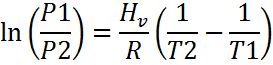In this equation, P1 and P2 are the vapor pressures of a material at temperatures T1 and T2, respectively. R is the ideal gas constant (8.314 J/(mol·K)) and Hv is the enthalpy of vaporization of the material, a number that you can look up for common materials. As expected, the equation shows that vapor pressure increases as temperature increases.

## Clausius-Clapeyron Equation Example

Let's look at an example using the Clausius-Clapeyron equation.

Given that the vapor pressure of water is 1 atm at its boiling point, 100°C (373 K), and that the enthalpy of vaporization of water is 40,700 J/mol, use the Clausius-Clapeyron Equation to determine the vapor pressure of water at 80°C (353 K).

The Clausius-Clapeyron equation can be rewritten in the following more-convenient form: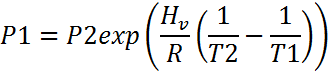We know that T1 = 353 K, T2 = 373 K, P2 = 1 atm, and Hv = 40,700 J/mol. Plugging these values into the equation, we get the following formula: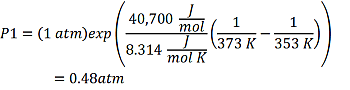Therefore, the vapor pressure of water at 80°C is 0.48 atm.

## Raoult's Law: Understanding Vapor Pressure in Mixtures

Sometimes we have to consider vapor pressure in a mixture of liquids. In the ideal case, the tendency of a molecule to escape will not change when it is mixed with another liquid and we can use Raoult's Law to determine the vapor pressure of the components.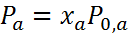To unlock this lesson you must be a Study.com Member.

Video Transcript

## What Is Vapor Pressure?

Water can take the form of a liquid, a solid (ice), or a gas (water vapor). Ice melts to form water, and water evaporates to form water vapor. Think about a water bottle like the one shown here:What's inside? You can see water and air, but what you may not have realized is that there is also water vapor. In a water bottle, a little bit of the water is constantly evaporating and becoming water vapor. At the same time, some of the water vapor is constantly condensing to become a liquid. At equilibrium, the amount of water evaporating is equal to the amount of water vapor condensing, so the amounts of water and water vapor are constant.

The water vapor exerts a pressure on the water bottle in the same way that air pumped into a tire exerts pressure on the tire. The pressure exerted by the water vapor is the vapor pressure. In more general terms, vapor pressure is the pressure exerted by a gas in equilibrium with the same material in liquid or solid form. Just as distance can be measured in a variety of units (miles, feet, kilometers, etc.), we can also measure vapor pressure with many different units (kPa, atm, bar, mm Hg, torr).

## The Clausius-Clapeyron Equation

Let's consider what happens if the temperature of the water bottle increases a little. The water molecules will have more energy to evaporate, so there will be a little more water vapor and a little less water in the bottle at equilibrium at this higher temperature. The amount of pressure exerted by the water vapor will also increase, meaning that the vapor pressure will increase. The Clausius-Clapeyron equation describes how temperature affects vapor pressure.In this equation, P1 and P2 are the vapor pressures of a material at temperatures T1 and T2, respectively. R is the ideal gas constant (8.314 J/(mol·K)) and Hv is the enthalpy of vaporization of the material, a number that you can look up for common materials. As expected, the equation shows that vapor pressure increases as temperature increases.

## Clausius-Clapeyron Equation Example

Let's look at an example using the Clausius-Clapeyron equation.

Given that the vapor pressure of water is 1 atm at its boiling point, 100°C (373 K), and that the enthalpy of vaporization of water is 40,700 J/mol, use the Clausius-Clapeyron Equation to determine the vapor pressure of water at 80°C (353 K).

The Clausius-Clapeyron equation can be rewritten in the following more-convenient form:We know that T1 = 353 K, T2 = 373 K, P2 = 1 atm, and Hv = 40,700 J/mol. Plugging these values into the equation, we get the following formula:Therefore, the vapor pressure of water at 80°C is 0.48 atm.

## Raoult's Law: Understanding Vapor Pressure in Mixtures

Sometimes we have to consider vapor pressure in a mixture of liquids. In the ideal case, the tendency of a molecule to escape will not change when it is mixed with another liquid and we can use Raoult's Law to determine the vapor pressure of the components.To unlock this lesson you must be a Study.com Member.

#### How is vapor pressure used in everyday life?

The pressure exerted by the vapor is utilized in pressure cookers, which are closed containers that trap the vapor in. Pressure cookers speed up the cooking of food by increasing the vapor pressure. It is done by sealing the cooker and letting vapors accumulate inside.

#### How to calculate vapor pressure?

The vapor pressure is the pressure exerted by vapor at equilibrium with its solid or liquid in a closed chamber. It can be calculated either by using Clausius-Clapeyron Equation or Raoult's Law.

• Raoult's Law is used when determining the vapor pressure of a component in a mixture.
• Clausius-Clapeyron Equation is used when determining the vapor pressure of a substance at different temperatures.

### Register to view this lesson

Are you a student or a teacher?

Back

### Resources created by teachers for teachers

Over 30,000 video lessons & teaching resources‐all in one place.Video lessonsQuizzes & WorksheetsClassroom IntegrationLesson Plans

I would definitely recommend Study.com to my colleagues. It’s like a teacher waved a magic wand and did the work for me. I feel like it’s a lifeline.

Jennifer B.
TeacherCreate an account to start this course today
Used by over 30 million students worldwide